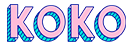# Voltage Sensor Module (0-25V) for Arduino

LKR144.00

6 in stock

or pay only LKR 48.00 now withSKU: L02P33 Category:

DESCRIPTION

• A simple but very useful module which uses a potential divider to reduce any input voltage by a factor of 5.
• This allows you to use the analog input of a microcontroller to monitor voltages much higher than it capable of sensing.
• For example, with a 0-5V analog input range you are able to measure a voltage up to 25V.
• The module also includes convenient screw terminals for easy and secure connection of wires.
• This module is based on the principle of resistive voltage divider design, can make the red terminal connector input voltage to 5 times smaller
• Arduino analog input voltages up to 5 v, the voltage detection module input voltage not greater than 5Vx5=25V (if using 3.3V systems, input voltage not greater than 3.3Vx5=16.5V).
• Arduino AVR chips have 10-bit AD, so this module simulates a resolution of 0.00489V (5V/1023), so the minimum voltage of input voltage detection module is 0.00489Vx5=0.02445V.

Features:

• Input voltage : 25v DC max
• Divider ratio : 5:1
• Output voltage : 5v DC (input/5 input*0.2)
• Output Current : 600mA (MAX)
• Voltage detection range : DC0.02445V – 25V
• Voltage analog resolution : 0.00489 V
• Size : 25mm x 13mm
• Output Interface :
“+ ” connected 5/3.3V
“-” connected GND
“s” connected Arduino AD pins

Weight 10 g

## See It Styled On Instagram